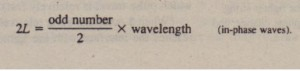# Equations for Thin Film Interference

Equations for Thin-Film Interference

In this chapter we have now seen three ways in which the phase difference between
two waves can change:
1. by reflection
2. by the waves traveling along paths of different lengths
3. by the waves traveling through media of different indexes of refraction
When light reflects from a thin film, producing the waves of rays ‘) and 1’2 shown  in , all three ways are involved. Let us consider them one by one. We first reexamine the two reflections in . At point a on the front interface. the incident wave (in air) reflects from the medium having the higher of  the two indexes of refraction, so the wave of reflected ray’) has its phase shiftedby 0.5 wavelength. At point b on the back interface, the incident wave reflects from
the medium (air) having  he lower of the two indexes of refraction, so the wave reflected there is not shifted in phase by the reflection, and thus neither is the portion of it that exits the film as ray ‘2’ We can organize this information with the first line in Table 36-1. It tells us that, so far, as a result of the reflection phase shifts, the0 waves of 1′) and 1’2 have a phase difference of 0.5 wavelength and thus are exactly out of phase. Now we must consider the path length difference U that occurs because the wave of ray ‘2 crosses the film twice. (This difference U is shown on the second
line in Table 36-1.) If the waves of r, and ‘2 are to be exactly in phase so that they  produce fully constructive interference, the path length U must cause an additional phase difference of 0.5, 1.5, 2.5, … wavelengths. Only then will the net phase difference be an integer number of wavelengths. Thus, for a bright film, we must have